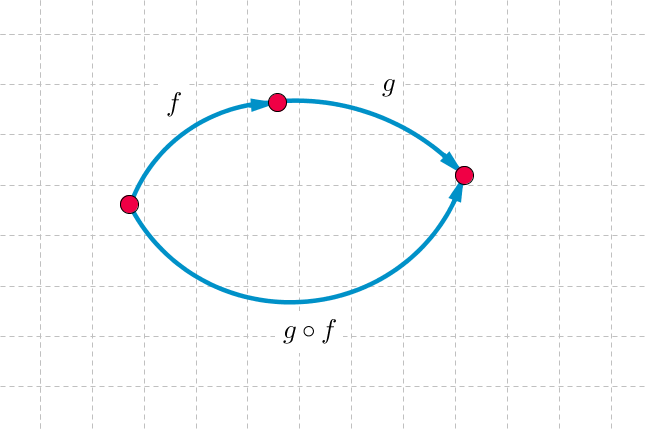3.22

## UNSW SydneyFirst f, then g.

# Composing functions

When we view graphs as functions, taking a given value of $\normalsize{x}$ and outputting a particular value of $\normalsize{y}$ according to a rule $\normalsize{y=f(x)}$, then an interesting new operation arises: composition.

In this step we look at composing functions rather generally, and give examples from the various kinds of linear, quadratic and inverse functions we have already studied.

## Composing functions

If $\normalsize{f}$ and $\normalsize{g}$ are functions, then the composition $\normalsize{g \circ f}$ is the function defined by:

It means that we first apply $\normalsize{f}$ to $\normalsize{x}$, and then apply $\normalsize{g}$ to $\normalsize{f(x)}$. This is one of those cases where it might be more natural to read right to left.

## Composition of linear functions

Let’s do a few examples involving simple linear functions. If $\normalsize{f(x)=x+3}$ and $\normalsize{g(x)=5x-1}$, then

Note that

and that this is different from $\normalsize{g \circ f}$.

We can also note that more generally the composition of two linear functions will be another linear function.

Q1 (E): If $\normalsize{h(x)=x-1}$ and $\normalsize{k(x)=3x+2}$, find $\normalsize (h \circ k)(x)$ and $\normalsize (k \circ h)(x)$.

However this is not so simple when we look at compositions of higher degree functions.

## Composition of quadratic functions

If $\normalsize{f(x)=x^2+1}$ and $\normalsize{g(x)=3x^2-x}$ then

In general we can see that the composition of two quadratic functions is going to be a quartic, or degree four, function.

However the composition of a quadratic function with a linear function will be a quadratic function.

Q2 (E): What is the composition $\normalsize{f \circ g}$ of the functions $\normalsize{f(x)=x^2+1}$ and $\normalsize{g(x)=3x-4}$?

Q3 (M): You buy a new kitchen table. Taxes are $\normalsize{15\%}$ and delivery is $\normalsize{\30}$. If the cost of the table is $\normalsize{x}$, then what is your total cost? In this question, there is a bit of an ambiguity, as we are not sure if taxes apply to delivery or not. Suppose we define functions $\normalsize{t(x)=1.15x}$ and $\normalsize{d(x)=x+30}$. Then what are $\normalsize{(t \circ d)(x)}$ and $\normalsize{(d \circ t)(x)}$? If taxes don’t apply to delivery costs, then which composite represents your total cost?

Q4 (C): Compositions are not always defined, so one has to be careful. Let $\normalsize{f(x)=-x^2}$ and $\normalsize{g(x)=\sqrt{x}}$. For which values of $\normalsize x$ are the compositions $\normalsize{(f \circ g)(x)}$ and $\normalsize{(g \circ f)(x)}$ defined?

Q5 (C): The morning operations $\normalsize t$ for “brushing teeth” and $\normalsize e$ for “eating breakfast” can be performed as $\normalsize t \circ e$ or $\normalsize e \circ t$. Daniel believes that the correct order of operations is $\normalsize t \circ e$ because it keeps teeth clean for longer. Norman reckons that breakfast tastes better when we choose the order $\normalsize e \circ t$. What do you think?

A1. The compositions are:

and

$\Large (k \circ h)(x) = k(x-1) = 3(x-1)+2= 3x-1$.

A2. The composition $\normalsize{f \circ g}$ is

A3. The compositions are:

If taxes don’t apply to delivery costs, then the total cost is given by $\normalsize{(d \circ t)(x)}.$

A4. The composition $\normalsize{(f \circ g)(x)}$ can be defined for all positive numbers $\normalsize{x}$, since

However, the composition

can be defined only for $\normalsize{x=0}$, since the value of the function $\normalsize{f}$ is otherwise negative and we cannot take the square root of a negative number.

A5. Let’s take a vote in the discussion.